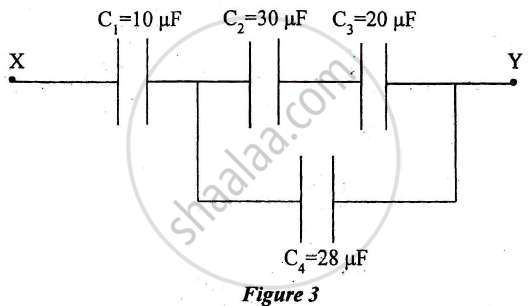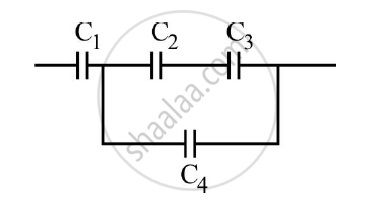Share

# Four Capacitors, C1, C2, C3 Andc4 Are Connected as Shown in Figure 3 Below. Calculate Equivalent Capacitance of the Circuit Between Points X . and Y. - ISC (Arts) Class 12 - Physics (Theory)

ConceptCapacitance of a Parallel Plate Capacitor with and Without Dielectric Medium Between the Plates

#### Question

Four capacitors, C1, C2, C3 andC4 are connected as shown in Figure 3 below. Calculate equivalent capacitance of the circuit between points X . and Y.#### Solution

C_2 and C_3 are in series and the resultant is in parallel with C_4

1/"C'" = 1/C_2 + 1/C_2 = 1/30 + 1/20

C' = (30xx 20)/(30 + 20) = 600/50 = 12 muF

C" = C' +   C_4 = 12 + 28 = 40muF

C_T =(C“ + C1)/(C” + C_1) = (40 xx 10)/(40 + 10)=  8muFIs there an error in this question or solution?

#### APPEARS IN

2014-2015 (March) (with solutions)
Question 3.1 | 3.00 marks

#### Video TutorialsVIEW ALL 

Solution Four Capacitors, C1, C2, C3 Andc4 Are Connected as Shown in Figure 3 Below. Calculate Equivalent Capacitance of the Circuit Between Points X . and Y. Concept: Capacitance of a Parallel Plate Capacitor with and Without Dielectric Medium Between the Plates.
S